Home MonkeyNotes Printable Notes Digital Library Study Guides Study Smart Parents Tips College Planning Test Prep Fun Zone Help / FAQ How to Cite New Title Request

Lagranges Mean Value Theorem

It is the generalization of the Rolles theorem. Let f (x) be any continuous function on [a , b] and differentiable in (a, b), then there exists at least one value of x = c Î (a, b) such that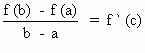i.e. f (b) - f (a) = ( b - a) f ' (c)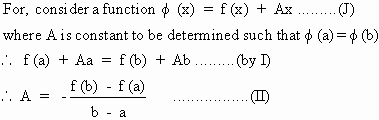Now f (x) satisfies all conditions of the Rolles theorem. Hence there exists at least one

x = c in (a , b) such that f ' (c) = 0

\ f ' (c) + A = 0 ........(from I)

\ A = - f ' (c) ............(from II), we get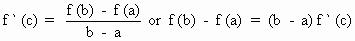Your browser does not support the IFRAME tag.

Geometrically - If the graph of y = f (x) is continuous, be drawn between x = a and x = b then A ( a, f (a)) and B (b , f (b)).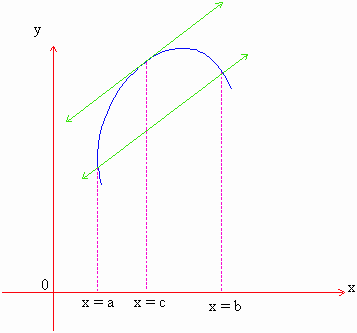\ Slope of the chord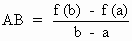by L.M.V. , this is equal to the slope of the tangent to y = f (x) at x = c i.e. f ' (c).

Hence the theorem states that there exists at least one tangent at x =c which is parallel to the chord AB.

Index

5.1 Tangent And Normal Lines
5.2 Angle Between Two Curves
5.3 Interpretation Of The Sign Of The Derivative
5.4 Locality Increasing Or Decreasing Functions 5.5 Critical Points
5.6 Turning Points
5.7 Extreme Value Theorem
5.8 The Mean-value Theorem
5.9 First Derivative Test For Local Extrema
5.10 Second Derivative Test For Local Extrema
5.11 Stationary Points
5.12 Concavity And Points Of Inflection
5.13 Rate Measure (distance, Velocity And Acceleration)
5.14 Related Rates
5.15 Differentials : Errors And ApproximationAll Contents Copyright © All rights reserved.
Further Distribution Is Strictly Prohibited.

1209286 PinkMonkey users are on the site and studying right now.